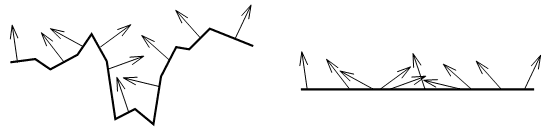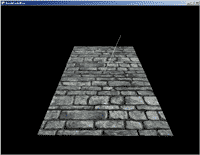# 5.13 凹凸映射：固定法线

## 解决方案## 工作原理

private VertexPositionTexture[] InitVertices()
{
VertexPositionTexture[] vertices = new VertexPositionTexture;
vertices = new VertexPositionTexture(new Vector3(-5, 0, 10), new Vector2(0, 2));
vertices = new VertexPositionTexture(new Vector3(-5, 0, -10), new Vector2(0, 0));
vertices = new VertexPositionTexture(new Vector3(5, 0, 10), new Vector2(1, 2));
vertices = new VertexPositionTexture(new Vector3(-5, 0, -10),new Vector2(0, 0));
vertices = new VertexPositionTexture(new Vector3(5, 0, -10), new Vector2(1, 0));
vertices = new VertexPositionTexture(new Vector3(5, 0, 10), new Vector2(1, 2));
return vertices;
} 

### XNA-to-HLSL变量

float4x4 xWorld;
float4x4 xView;
float4x4 xProjection;
float3 xLightDirection;

Texture xTexture;
sampler TextureSampler = sampler_state
{
texture = <xTexture> ;
magfilter = LINEAR;
minfilter = LINEAR;
mipfilter=LINEAR;
};

Texture xBumpMap;
sampler BumpMapSampler = sampler_state
{
texture = <xBumpMap> ;
magfilter = LINEAR;
minfilter = LINEAR;
mipfilter=LINEAR;
};

struct SBMVertexToPixel
{
float4 Position : POSITION;
float2 TexCoord : TEXCOORD0;
};

struct SBMPixelToFrame
{
float4 Color : COLOR0;
};

SBMVertexToPixel SBMVertexShader(float4 inPos: POSITION0, float2 inTexCoord: TEXCOORD0)
{
SBMVertexToPixel Output = (SBMVertexToPixel)0;
float4x4 preViewProjection = mul(xView, xProjection);
float4x4 preWorldViewProjection = mul(xWorld, preViewProjection);
Output.Position = mul(inPos, preWorldViewProjection);
Output.TexCoord = inTexCoord;
return Output;
} 

SBMPixelToFrame SBMPixelShader(SBMVertexToPixel PSIn) : COLOR0
{
SBMPixelToFrame Output = (SBMPixelToFrame)0;
float3 bumpMapColor = tex2D(BumpMapSampler, PSIn.TexCoord).rbg;
float3 normalFromBumpMap = (bumpMapColor - 0.5f)*2.0f;
float lightFactor = dot(-normalize(normalFromBumpMap), normalize(xLightDirection));
float4 texColor = tex2D(TextureSampler, PSIn.TexCoord);
Output.Color = lightFactor*texColor;
return Output;
} 

### 定义Technique

technique SimpleBumpMapping
{
pass Pass0
{
}
} 

## 代码

protected override void Draw(GameTime gameTime)
{
device.Clear(ClearOptions.Target | ClearOptions.DepthBuffer, Color.Black, 1, 0);

//set effect parameters
effect.CurrentTechnique = effect.Techniques["SimpleBumpMapping"];
effect.Parameters["xWorld"].SetValue(Matrix.Identity);
effect.Parameters["xView"].SetValue(fpsCam.ViewMatrix);
effect.Parameters["xProjection"].SetValue(fpsCam.ProjectionMatrix);
effect.Parameters["xTexture"].SetValue(myTexture);
effect.Parameters["xBumpMap"].SetValue(myBumpMap);
effect.Parameters["xLightDirection"].SetValue(lightDirection);

//render two triangles
effect.Begin();
foreach (EffectPass pass in effect.CurrentTechnique.Passes)
{
pass.Begin();
device.VertexDeclaration = myVertexDeclaration;
device.DrawUserPrimitives<VertexPositionTexture>(PrimitiveType.TriangleList, vertices, 0, 2);
pass.End();
}
effect.End();
base.Draw(gameTime);
}2006 - 2023，推荐分辨率1024*768以上，推荐浏览器Chrome、Edge等现代浏览器，截止2021年12月5日的访问次数：1872万9823 站长邮箱